# Simplicial object in a category

(diff) ← Older revision | Latest revision (diff) | Newer revision → (diff)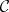A contravariant functor(or, equivalently, a covariant functor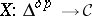) from the category, whose objects are ordered sets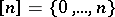,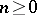, and whose morphisms are non-decreasing mappings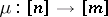, into the category. A covariant functor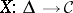(or, equivalently, a contravariant functor) is called a co-simplicial object in.

The morphisms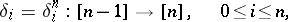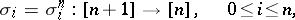ofgiven by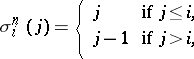generate all the morphisms of, so that a simplicial objectis determined by the objects,(called the-fibres or-components of the simplicial object), and the morphisms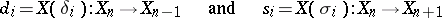(called boundary operators and degeneracy operators, respectively). In caseis a category of structured sets, the elements ofare usually called the-dimensional simplices of. The mappings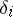and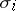satisfy the relations(*)

and any relation between these mappings is a consequence of the relations (*). This means that a simplicial objectcan be identified with a systemof objects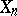,, ofand morphisms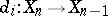and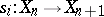,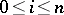, satisfying the relations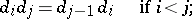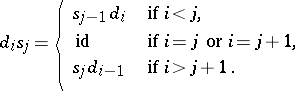Similarly, a co-simplicial objectcan be identified with a systemof objects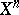,(-co-fibres) and morphisms,(co-boundary operators), and,(co-degeneracy operators), satisfying the relations (*) (with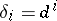,).

A simplicial mappingbetween simplicial objects (in the same category) is a transformation (morphism) of functors frominto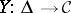, that is, a family of morphisms,, ofsuch that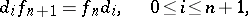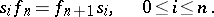The simplicial objects ofand their simplicial mappings form a category, denoted by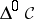.

A simplicial homotopy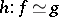between two simplicial mappingsbetween simplicial objects in a categoryis a family of morphisms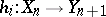,, ofsuch that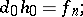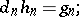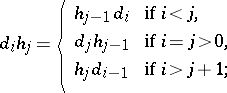On the basis of this definition one can reproduce in essence the whole of ordinary homotopy theory in the category, for any category. In the case of the category of sets or topological spaces, the geometric realization functor (see Simplicial set) carries this "simplicial" theory into the usual one.

Examples of simplicial objects are a simplicial set, a simplicial topological space, a simplicial algebraic variety, a simplicial group, a simplicial Abelian group, a simplicial Lie algebra, a simplicial smooth manifold, etc.

Every simplicial Abelian group can be made into a chain complex with boundary operator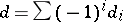.

How to Cite This Entry:
Simplicial object in a category. Encyclopedia of Mathematics. URL: http://encyclopediaofmath.org/index.php?title=Simplicial_object_in_a_category&oldid=12531
This article was adapted from an original article by S.N. MalyginM.M. Postnikov (originator), which appeared in Encyclopedia of Mathematics - ISBN 1402006098. See original article